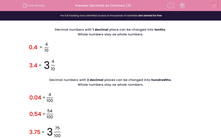# Decimals as Fractions (3)

In this worksheet, students must read and write decimal numbers up to thousandths as fractions or mixed numbers.This content is premium and exclusive to EdPlace subscribers.Key stage:  KS 2

Curriculum topic:   Maths and Numerical Reasoning

Curriculum subtopic:   Decimals

Difficulty level:#### Worksheet Overview

Decimal numbers with 1 decimal place can be changed into tenths.

Whole numbers stay as whole numbers.

 0.4 = 4 10

 3.4 = 3 4 10

Decimal numbers with 2 decimal places can be changed into hundredths.

Whole numbers stay as whole numbers.

 0.04 = 4 100

 0.54 = 54 100

 3.75 = 3 75 100

Decimal numbers with 3 decimal places can be changed into thousandths.

Whole numbers stay as whole numbers.

 0.004 = 4 1000

 0.054 = 54 1000

 3.715 = 3 715 1000

The reverse is also true.

Example 1

Write the following fraction as a decimal.

 5 1000

5 thousandths is 0.005 with 3 decimal places.

(Use zeros to fill in the spaces.)

Example 2

Write the following mixed number as a decimal.

 4 15 1000

15 thousandths is 0.015 with 3 decimal places.

The 4 is a whole number and stays the same.